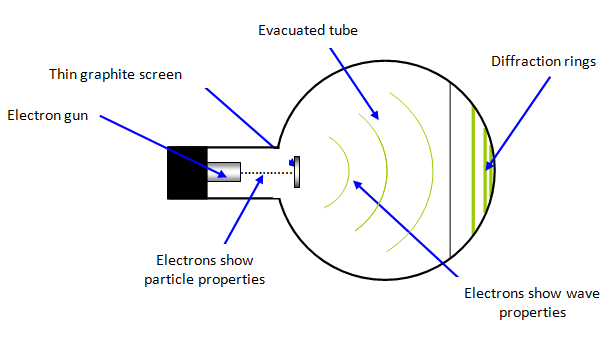Electron diffraction

If the electron has wave properties, then it ought to be possible to observe these: electrons should therefore show the characteristics of waves such as interference and diffraction. This is clearly a difficult concept if we are used to thinking of the electron as a particle. We must, however, abandon the out-of-date idea that the electron, or in fact any particle, always behaves like a solid object!The very small wavelength of electrons means that the obstacles used to diffract them must also be very small, and as with X-rays it was the atomic lattice that was eventually found to be suitable.

The diffraction of electrons was first shown by Davisson and Germer in 1927 and it can now be observed easily in schools with the correct apparatus.

A beam of electrons is accelerated in an electron gun to a potential of between 3500 V and 5000 V and then allowed to fall on a very thin sheet of graphite (see diagram above). The electrons diffract from the carbon atoms and the resulting circular pattern on the screen (see diagrams below) is very good evidence for the wave nature of the electrons.

The diffraction pattern observed on the screen is a series of concentric rings. This is due to the regular spacing of the carbon atoms in different layers in the graphite. However since the graphite layers overlay each other in an irregular way the resulting diffraction pattern is circular. It is an example of Bragg scattering.Low accelerating voltage High accelerating voltage

Wavelength of an electron (λ) = h/p     where p is the momentum of the electron

Example problems
Find the wavelength of an electron moving at 3x107 ms-1. (mass of electron = 9x10-31 kg).

Wavelength (λ) = h/p = h/mv = 6.63x10-34/[9x10-31x3x107] = 2.46x10-11 m = 0.0246 nm

If the voltage on the anode is increased the energy of the electrons is increased, and the diameter of a given ring gets less. This is exactly similar to the observation that blue light is diffracted less strongly than red light, which arises because the wavelength of blue light is smaller than that of red and hence its energy is larger.

Wavelength of an electron (λ) accelerated through a p.d of V volts = 12.27x10-10/V1/2

At very high energies the electrons are diffracted from the nuclei of the material – so probing deeper into matter! At these energies we must take relativistic effects into account and the formula becomes:

Wavelength of an electron (λ) = h/p ~ hc/E     where E is the electron energy

Example problems
Calculate the wavelength of an electron accelerated through a potential difference of:
(a) 10 keV and (b) 300 MeV.

(a) λ = 12.35x10-10/V1/2 = 1.25 x 10-11 m.
(b) λ = hc/E = 4.14 x 10-15 m   - similar to the dimensions of the atomic nucleus!

NB In example (b) the energy must be converted to joules (300 MeV = 300x106x1.6x10-19 J)

Problems
1. Calculate the wavelength of an electron accelerated through a p.d. of:
(a) 15 000 V in a colour television tube
(b) 200 000 V in an electron microscope
(c) 10 MV in a linear accelerator.

2. What would be the velocity of an electron with a wavelength of 0.1 nm?
3. A sodium atom with a mass of 23 u emits a photon with a wavelength of 589.6 nm. What is the recoil velocity of the sodium atom?schoolphysics: Electron diffraction animation

To see an animation of electron diffraction click on the animation link.

A VERSION IN WORD IS AVAILABLE ON THE SCHOOLPHYSICS USB